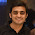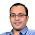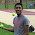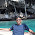Apr 20, 2013

Penny Roll Puzzle

Source: Quantnet

Problem:
Roll a penny around another fixed penny in the center with edges in close contact. After moving half circle around the center penny, you will find the penny in motion has rotated 360 degrees.
Why?

Update (29/06/2013):
Solution posted by Sanket Patel and Suyash Jain (IITB Mech 2008 Aumnus, Ex-Credit Suisse Analyst, Ex-Deutsche Bank Analyst) in comments!

1.radius of fixed penny is double than that of moving penny.

1.The radii of the two coins are same. Sorry

2.Consider a straight line. Start with two coins on the opposite half planes with similar orientations adjacent to each other and they roll in the same linear direction (along the line). Both the coins will make respective circles. Now, warp this line around the first coin. The first coin remains stationary, but because of warping of the line, the rotation of first coin gets added to the second one and hence the full circle.

1.Interesting

3.Consider an alternate scenario.
Let 2 coins be rolling across each other in opposite directions at a speed of 1 rotation per minute. A person sitting on one of the coins at the initial point of contact sees the other coin passing by at 2 rotations per second. Also exactly 1 minute past the initial moment, they will have reached their initial configuration.
Now stop 1 coin and roll the other coin over its circumference so that the coins reach their initial configuration in exactly 1 minute so that nothing will change for the person sitting on one of the coins. He still must see the other coin rolling past at 2 rotations per minute which is only possible if the only rolling coin is rolling at 2 rotations per minute. So after exactly 1 minute, the coin will have rotated 2 rotations to return to the initial configuration.
Hence 1 rotation about other coin = 2 rotations about own axis. divide by half and we have the result :)

1.I am not sure if that is correct or wrong. The approach for sure is confusing. Simpler the better :) Please have a look at the solution

4.The distance travelled by centre of outer penny for moving half circle around the fixed penny= Pie* (R+R) = 2*Pie*R, where R is the radius.
Now the problem can be seen as a rotation of ring on a plane with distance to be covered by the centre of ring = 2*pie*R
Assuming that it's a case of pure rolling: Distance covered by centre = Angle of rotation * Radius => Angle of rotation = 2*pie, which is 360* rotation.

Cheers!

1.Perfect. Thanks

2.Why is the distance pie *(R+R)? Outer penny moved half circle so distance is only pie*R, right?

3.The radius of rotation of the centre of outer penny is R+R.

5.On a related note, this is related to the difference between the difference of synodic vs. sidereal days in astronomy. The point is that in one complete "revolution" of a "planet" around its "sun", there is one fewer synodic or "solar" day than the number of sidereal days (the total number of rotations with respect to the "fixed" stars). For earth, for example, the mean solar day is 24h, while the mean sidereal days is about 23h56min. This corresponds to the usually quoted figure of 365.24 "days" in a solar years: the number of sidereal days is one more at 366.24.

6.The point of contact has 2*w angular velocity in frame of second coin thus angular displacement will also be double.

Fraction Brainteaser

Source: Sent to me by Gaurav Sinha Problem: Siddhant writes a Maths test and correctly answers 5 out of 6 Arithmetic questions and 20...